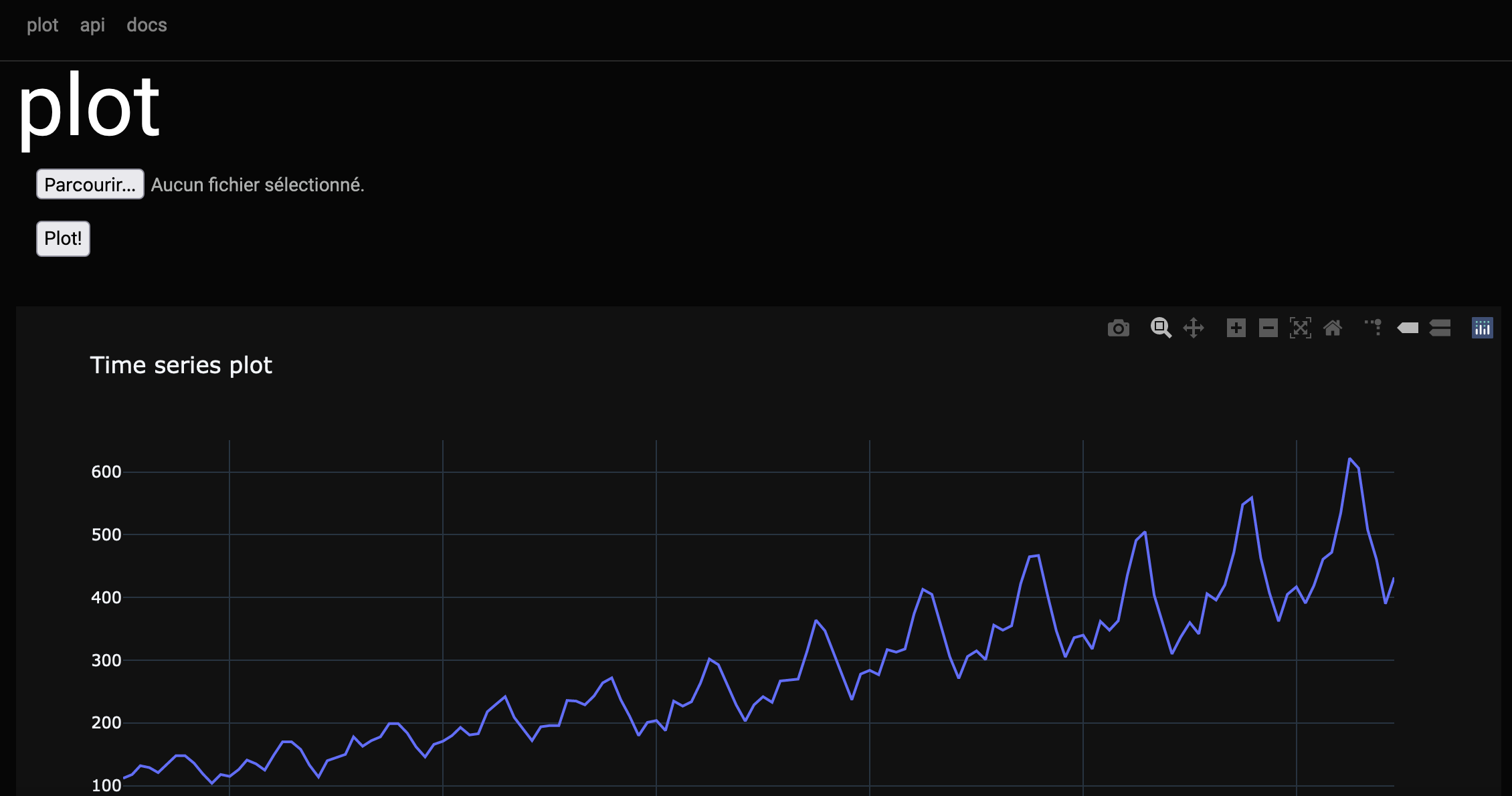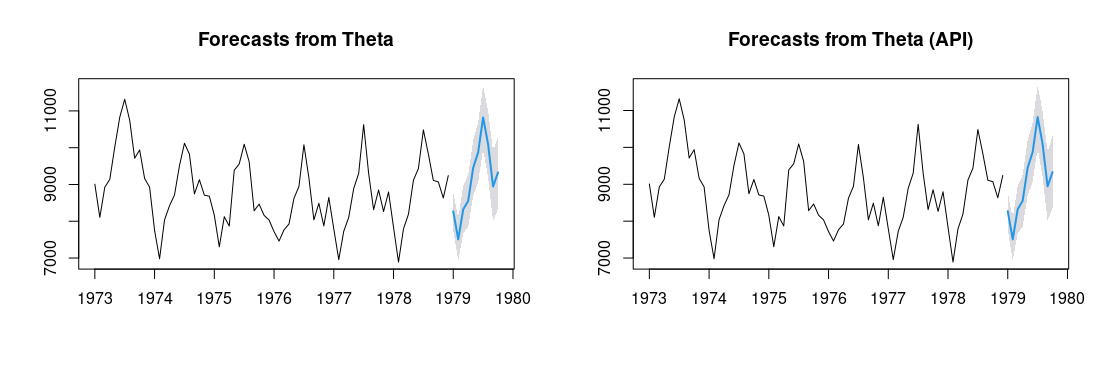Content

• 0 - Intro
• 1 - Create an account
• 2 - Get a token for authentication
• 3 - Requests for forecasts
• 4 - On model calibration and cross-validation

## 0 - Intro

In this post, I’ll describe an (work-in-progress) Application Programming Interface (API) for time series forecasting. An API is a system that can receive requests from your computer, to carry out given tasks on given resources, and return a response. This type of system is programming-language-agnostic. That means: it can be used with Python, JavaScript, PHP, R, Go, C#, Ruby, Rust, Java, MATLAB, Julia, and any other programming language speaking http. And therefore, it could be relatively easily integrated into existing workflows for uncertainty forecasting. I’ve used the following tools for building it:

The application is here:

https://techtonique2.herokuapp.com/In the homepage (“/”), you can plot a time series by uploading a csv file, and pushing the button “Plot!”. Some examples of input files are stored on GitHub, at https://github.com/Techtonique/datasets/tree/main/time_series/univariate. Hover your cursor over the graph to see the options available, like downloading as png, zooming in and out, etc. Let’s describe the API now.

## 1 - Create an account:

Using curl

curl -X POST -H "Content-Type: application/json" -d '{"username":"tester_curl@example.com","password":"pwd"}' https://techtonique2.herokuapp.com/api/users


If you want to translate these commands from curl to your favorite programming language (Python, JavaScript, PHP, R, Go, C#, Ruby, Rust, Elixir, Java, MATLAB, Dart, CFML, Ansible URI, Strest), you can simply use the following website: https://curlconverter.com/. Of course, it’s important to choose a better password!

Using Python

In the near future, there will be a user-friendly Python package encapsulating these steps.

import requests

# 'Content-Type': 'application/json',
}

json_data = {
}



Using R

In the near future, there will be a user-friendly R package encapsulating these steps.

require(httr)

Content-Type = 'application/json'
)

res <- httr::POST(url = 'https://techtonique2.herokuapp.com/api/users',

print(res)


Now that you have an account, you’ll need a token to obtain time series forecasts. The username and password could be used for that purpose, but it’s better to avoid sending them in every request. In any case, make sure that you’re always sending requests to https:// and not http://.

## 2 - Get a token for authentication

Using curl

curl -u tester_curl@example.com:pwd -X GET https://techtonique2.herokuapp.com/api/token


If you want to translate these commands from curl to your favorite programming language (Python, JavaScript, PHP, R, Go, C#, Ruby, Rust, Elixir, Java, MATLAB, Dart, CFML, Ansible URI, Strest), you can simply use the following website: https://curlconverter.com/.

Using Python

In the near future, there will be a user-friendly Python package encapsulating these steps.

response_token = requests.get('https://techtonique2.herokuapp.com/api/token',
auth=('tester_py@example.com', 'pwd'))

token = response_token.json()['token']

print("\n")
print(f"token: {token}")


Using R

In the near future, there will be a user-friendly R package encapsulating these steps.

res_token <- httr::GET(url = 'https://techtonique2.herokuapp.com/api/token',
httr::authenticate('tester_r@example.com', 'python22orpython33'))

print(res_token)

(token <- httr::content(res_token)$token)  ## 3 - Requests for forecasts We want to obtain 10 months-ahead forecasts for the number of accidental Deaths in the US from 1973 to 1978 , and a confidence level of 95% for prediction intervals. The forecasting method is Theta from  and , winner of the M3 competition. The token from section 2 (valid for 5 minutes) will be used here for authentication. You should read the API’s documentation to understand each forecasting model’s parameters. Using curl curl -u eyJhbGciOiJIUzI1NiIsInR5cCI6IkpXVCJ9.eyJpOCsOC8-I:x -F 'file=@/Users/t/Documents/datasets/time_series/univariate/USAccDeaths.csv' "https://techtonique2.herokuapp.com/api/theta?h=10&level=95"  If you want to translate these commands from curl to your favorite programming language (Python, JavaScript, PHP, R, Go, C#, Ruby, Rust, Elixir, Java, MATLAB, Dart, CFML, Ansible URI, Strest), you can simply use the following website: https://curlconverter.com/. eyJhbGciOiJIUzI1NiIsInR5cCI6IkpXVCJ9.eyJpOCsOC8-I is a simplified example of the type of token which can be obtained in step 2. The csv file sent in the request must be stored on your computer. Examples of such files can be found here. Using Python In the near future, there will be a user-friendly Python package encapsulating these steps. params = { 'h': '10', 'level': '95', } files = { 'file': open('./USAccDeaths.csv', 'rb') # File available at https://github.com/Techtonique/datasets/tree/main/time_series/univariate } response_forecast = requests.post('https://techtonique2.herokuapp.com/api/theta', files=files, params=params, auth=(token, 'x')) print(response_forecast.json())  Using R In the near future, there will be a user-friendly R package encapsulating these steps. params = list( h = '10', # horizon level = '95' # level of confidence for prediction intervals ) files = list( file = upload_file('./USAccDeaths.csv') # File available at https://github.com/Techtonique/datasets/tree/main/time_series/univariate ) ptm <- proc.time() res_forecast <- httr::POST(url = 'https://techtonique2.herokuapp.com/api/theta', query = params, body = files, encode = 'multipart', httr::authenticate(token, 'x')) proc.time() - ptm list_res <- httr::content(res_forecast) # Plot results # 1 - Results From R package forecast ----- require(forecast) (forecast_object_R <- forecast::thetaf(USAccDeaths, h=10, level = 95)) # 2 - Results From a Python implementation (in the API) ----- h <- length(list_res$ranges)

forecast_object_api <- list()
forecast_object_api$mean <- forecast_object_api$upper <- forecast_object_api$lower <- ts(rep(0, h), start = start(forecast_object_R$mean),
frequency = frequency(forecast_object_R$x)) for (i in 1:h) { forecast_object_api$mean[i] <- list_res$averages[[i]][] forecast_object_api$lower[i] <- list_res$ranges[[i]][] forecast_object_api$upper[i] <- list_res$ranges[[i]][] } forecast_object_api$x <- forecast_object_R$x forecast_object_api$method <- paste0(forecast_object_R$method, " (API)") forecast_object_api$level <- forecast_object_R\$level
forecast_object_api <- structure(forecast_object_api, class = "forecast")

print(forecast_object_api)
print(forecast_object_R)

# graphs

par(mfrow=c(1, 2))
plot(forecast_object_R)
plot(forecast_object_api)## 4 - On model calibration and cross-validation

Each model in the API has 2 additional parameters that we haven’t discussed yet:

• start_training: Start training index for cross-validation
• n_training: Size of training set window for cross-validation

Both of these parameters are to be used in a loop, in your favorite programming language, when you want to compare models’ performance, or tune their hyperparameters (model calibration). You’d code a loop (with 3-seconds delays between each API call in the loop, because you’re nice!) in which:

• start_training is incremented of 1 at each iteration, and n_training remains constant.
• n_training is incremented of 1 at each iteration, and start_training remains constant.

More on this (cross-validation and model calibration) in a future post. Stay tuned.

 Assimakopoulos, V., & Nikolopoulos, K. (2000). The theta model: a decomposition approach to forecasting. International journal of forecasting, 16(4), 521-530.

 Hyndman, R. J., & Billah, B. (2003). Unmasking the Theta method. International Journal of Forecasting, 19(2), 287-290.